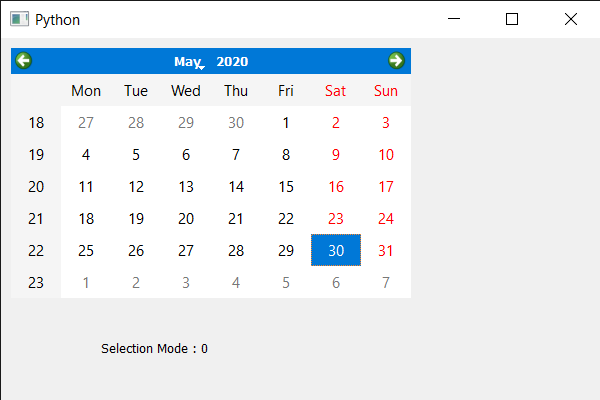# PyQt5 QCalendarWidget – Getting the Selection mode

• Last Updated : 25 Nov, 2021

In this article, we will see how we can get the selection mode of the QCalendarWidget. Selection mode is basically the quality of the calendar how date is selected. There are two modes available for the QCalendarWidget first is the NoSelection mode its value is 0 and in this mode no date can be selected. And other is the SingleSelection its is the default mode and its value is 1, in this mode at a time single date can be selected. We use setSelectionMode method to set the mode to the QCalendarWidget object.

In order to do this we will use selectionMode method with the QCalendarWidget object.
Syntax : calendar.selectionMode()
Argument : It takes no argument
Return : It returns selection mode object but when printed it shows associated value with the mode.

Below is the implementation

## Python3

 `# importing libraries``from` `PyQt5.QtWidgets ``import` `*``from` `PyQt5 ``import` `QtCore, QtGui``from` `PyQt5.QtGui ``import` `*``from` `PyQt5.QtCore ``import` `*``import` `sys`  `class` `Window(QMainWindow):` `    ``def` `__init__(``self``):``        ``super``().__init__()` `        ``# setting title``        ``self``.setWindowTitle(``"Python "``)` `        ``# setting geometry``        ``self``.setGeometry(``100``, ``100``, ``600``, ``400``)` `        ``# calling method``        ``self``.UiComponents()` `        ``# showing all the widgets``        ``self``.show()` `    ``# method for components``    ``def` `UiComponents(``self``):` `        ``# creating a QCalendarWidget object``        ``calendar ``=` `QCalendarWidget(``self``)` `        ``# setting geometry to the calendar``        ``calendar.setGeometry(``10``, ``10``, ``400``, ``250``)` `        ``# setting selection mode``        ``calendar.setSelectionMode(QCalendarWidget.NoSelection)` `        ``# creating a label``        ``label ``=` `QLabel(``self``)` `        ``# setting geometry to the label``        ``label.setGeometry(``100``, ``280``, ``250``, ``60``)` `        ``# making label multi line``        ``label.setWordWrap(``True``)` `        ``# getting the selection mode``        ``value ``=` `calendar.selectionMode()` `        ``# setting text to the label``        ``label.setText(``"Selection Mode : "` `+` `str``(value))`    `# create pyqt5 app``App ``=` `QApplication(sys.argv)` `# create the instance of our Window``window ``=` `Window()` `# start the app``sys.exit(App.``exec``())`

Output :My Personal Notes arrow_drop_up